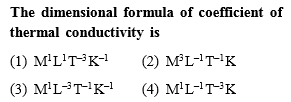Concept Videos :-

#1 | Basic Concepts & Examples
#2 | Dimensional Analysis : Remaining

Concept Questions :-

Dimensions
Complete Question Bank + Test Series
Complete Question Bank

Difficulty Level: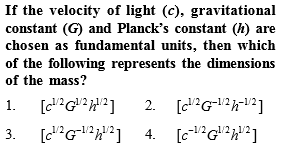Concept Videos :-

#1 | Basic Concepts & Examples
#2 | Dimensional Analysis : Remaining

Concept Questions :-

Dimensions
Complete Question Bank + Test Series
Complete Question Bank

Difficulty Level: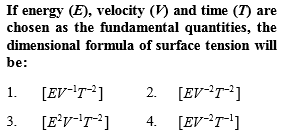Concept Videos :-

#1 | Basic Concepts & Examples
#2 | Dimensional Analysis : Remaining

Concept Questions :-

Dimensions
Complete Question Bank + Test Series
Complete Question Bank

Difficulty Level: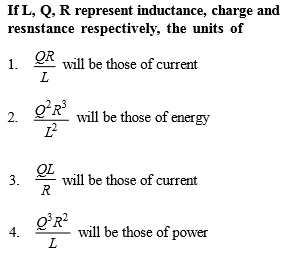[Only for Dropper batch]

Concept Videos :-

#1 | Basic Concepts & Examples
#2 | Dimensional Analysis : Remaining

Concept Questions :-

Dimensions
Complete Question Bank + Test Series
Complete Question Bank

Difficulty Level: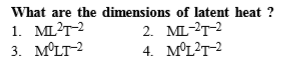Concept Videos :-

#1 | Basic Concepts & Examples
#2 | Dimensional Analysis : Remaining

Concept Questions :-

Dimensions
Complete Question Bank + Test Series
Complete Question Bank

Difficulty Level: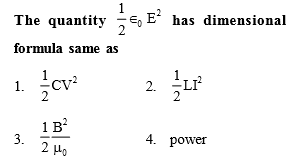[Only for dropper batch]

Concept Videos :-

#1 | Basic Concepts & Examples
#2 | Dimensional Analysis : Remaining

Concept Questions :-

Dimensions
Complete Question Bank + Test Series
Complete Question Bank

Difficulty Level: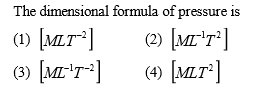Concept Videos :-

#1 | Basic Concepts & Examples
#2 | Dimensional Analysis : Remaining

Concept Questions :-

Dimensions
Complete Question Bank + Test Series
Complete Question Bank

Difficulty Level:

Light year is a unit of

(1) Time

(2) Mass

(3) Distance

(4) Energy

Concept Videos :-

#1 | Basic Concepts & Examples
#2 | Dimensional Analysis : Remaining

Concept Questions :-

Dimensions
Complete Question Bank + Test Series
Complete Question Bank

Difficulty Level:

The magnitude of any physical quantity

(1) Depends on the method of measurement

(2) Does not depend on the method of measurement

(3) Is more in SI system than in CGS system

(4) Directly proportional to the fundamental units of mass, length and time

Concept Videos :-

#1 | Basic Concepts & Examples
#2 | Dimensional Analysis : Remaining

Concept Questions :-

Dimensions
Complete Question Bank + Test Series
Complete Question Bank

Difficulty Level:

Which of the following is not equal to watt ?

(1) Joule/second

(2) Ampere $×$ volt

(3) (Ampere)2 $×$ ohm

(4) Ampere/volt

Concept Videos :-

#1 | Basic Concepts & Examples
#2 | Dimensional Analysis : Remaining

Concept Questions :-

Dimensions